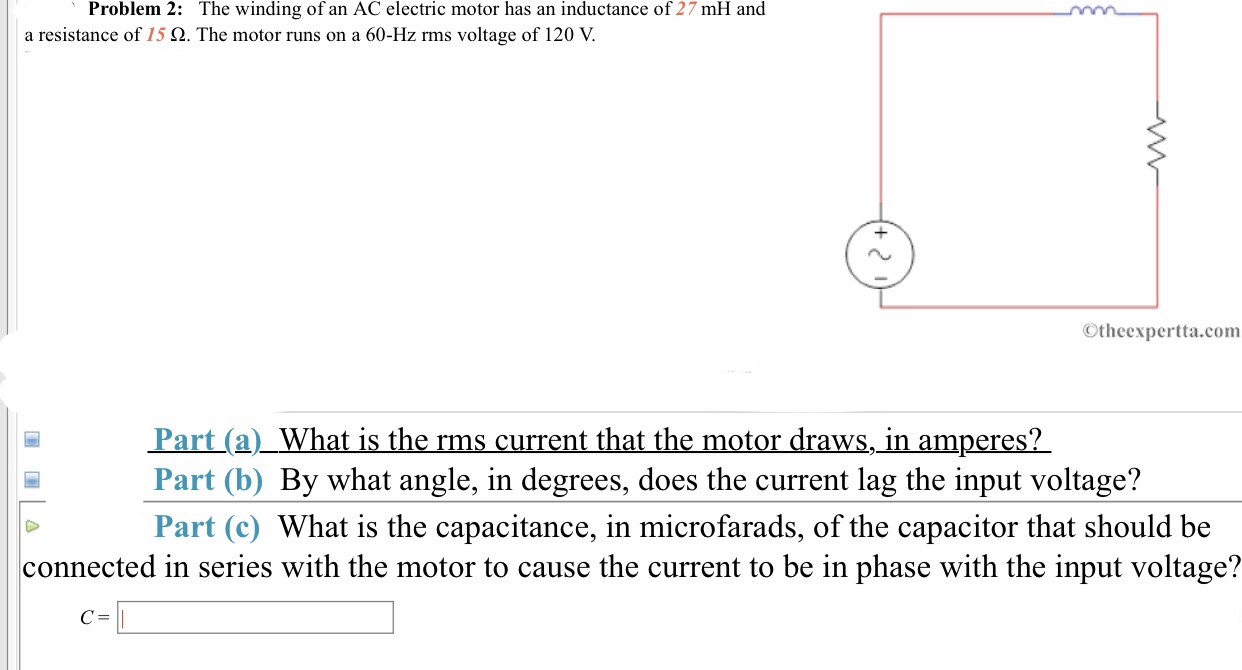# Problem 2:The winding of an AC electric motor has an inductance of 27 mH and60-Hz rms voltage of 120 V.a resistance of 15 Ω. The motor runs onOtheexpertta.comPart(a)_What is the rms current that the motor draws, in amperes?Part (b) By what angle, in degrees, does the current lag the input voltage?Part (c) What is the capacitance, in microfarads, of the capacitor that should beconnected in series with the motor to cause the current to be in phase with the input voltage?

Question
88 viewshelp_outlineImage TranscriptioncloseProblem 2: The winding of an AC electric motor has an inductance of 27 mH and 60-Hz rms voltage of 120 V. a resistance of 15 Ω. The motor runs on Otheexpertta.com Part(a)_What is the rms current that the motor draws, in amperes? Part (b) By what angle, in degrees, does the current lag the input voltage? Part (c) What is the capacitance, in microfarads, of the capacitor that should be connected in series with the motor to cause the current to be in phase with the input voltage? fullscreen
check_circle

Step 1

Given: Inductance, L = 27 mH

Resistance, R = 15 ohm

Frequency, f = 60 Hz

Voltage, V = 120 V

Find: (a) The rms current that the motor draws.

(b) By what angle, does the current lag the input voltage.

(c) The capacitance of the capacitors that should be connected in series with the motor to cause the current to be in phase with input voltage

Step 2

First calculate the inductive resistance of the inductor as:

Step 3

Now, calculate impedence, Z  ...

### Want to see the full answer?

See Solution

#### Want to see this answer and more?

Solutions are written by subject experts who are available 24/7. Questions are typically answered within 1 hour.*

See Solution
*Response times may vary by subject and question.
Tagged in

### Current Electricity